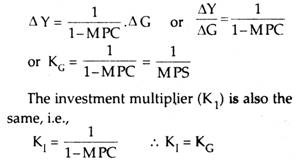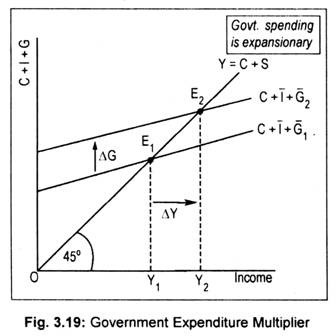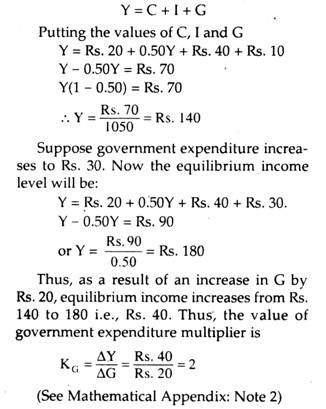# Government Expenditure Multiplier: G-Multiplier (With Diagram)

Like private investment, an increase in government spending results in an increase in national income.

Thus, its effect on national income is expansionary. There is a limit to private investment. Thus, to stimulate income the gap has to be filled up by government expenditure.

However, the increase in income is greater than the increase in government spending. The impact of a change in income following a change in government spending is called government expenditure multiplier, symbo­lised by kG.

The government expenditure multiplier is, thus, the ratio of change in income (∆Y) to a change in government spending (∆G). Thus,

KG = ∆Y/∆G and ∆Y = KG. ∆G

In other words, an autonomous increase in government spending generates a multiple expansion of income. How much income would expand depends on the value of MPC or its reciprocal, MPS. The formula for KG is the same as the simple investment multiplier, represented by KI.

Its formula (i.e., KG) is:The impact of a change in government spending is illustrated graphically in Fig. 3.19 where C + 1 + G1 is the initial aggregate demand schedule. E1 is the initial equilibrium point and the corresponding level of income is, thus, OY1If the government plans to spend more, aggregate demand schedule would then shift to C + I + g2. As this line cuts the 45° line at E2, the new equilibrium level of income, thus, rises to OY2— an amount larger than the initial one. It is clear from Fig. 3.19 that the increase in income (∆Y) is larger than the increase in government spending (∆G).The reason behind this expansionary effect of government spending on income is that the increase in public expenditure constitutes an increase in income, thereby triggering successive increases in consumption, which also constitutes increase in income. However, greater the MPC, greater will be the increase in income.

Mathematical Example of Govern­ment Expenditure Multiplier: